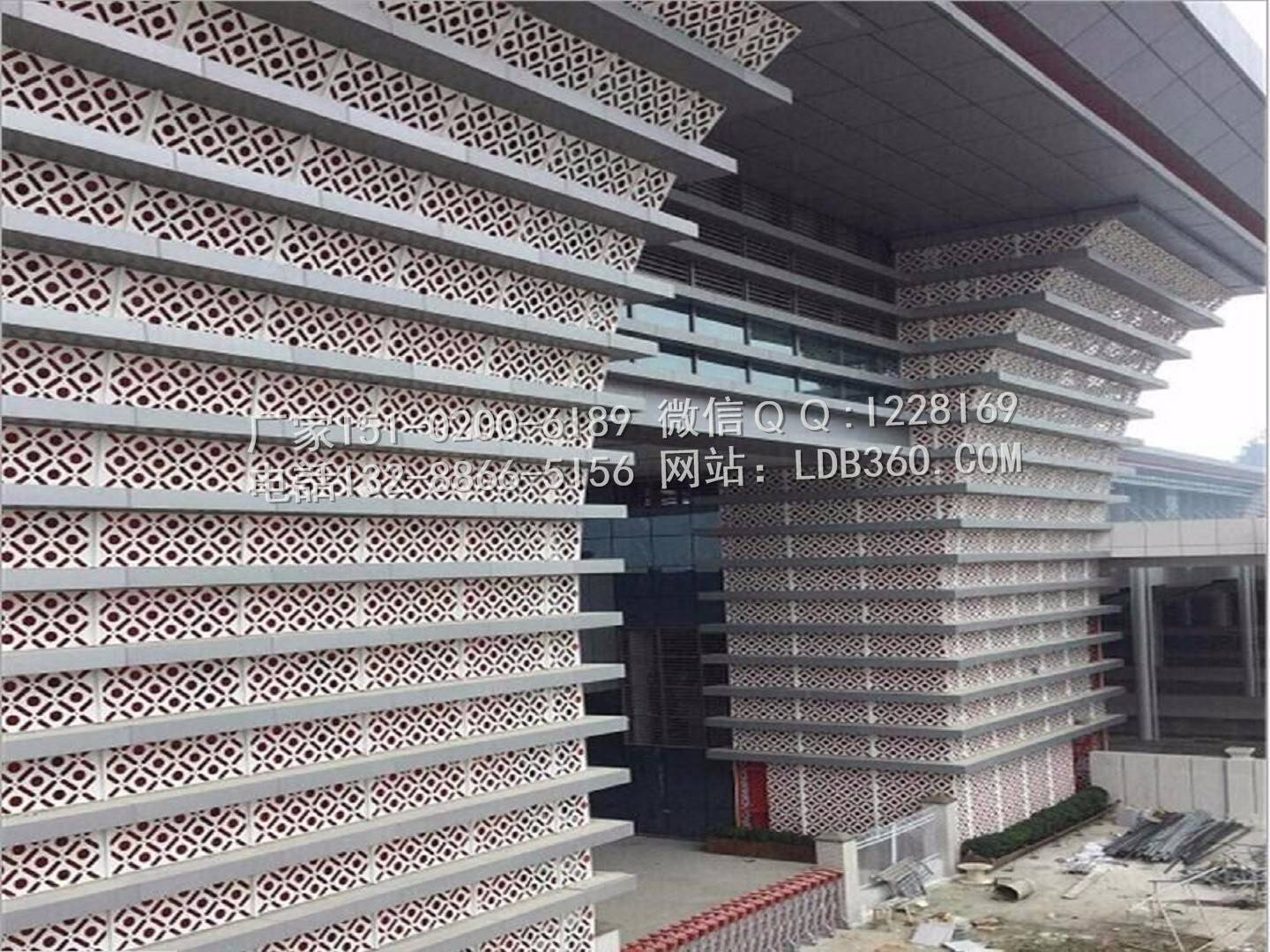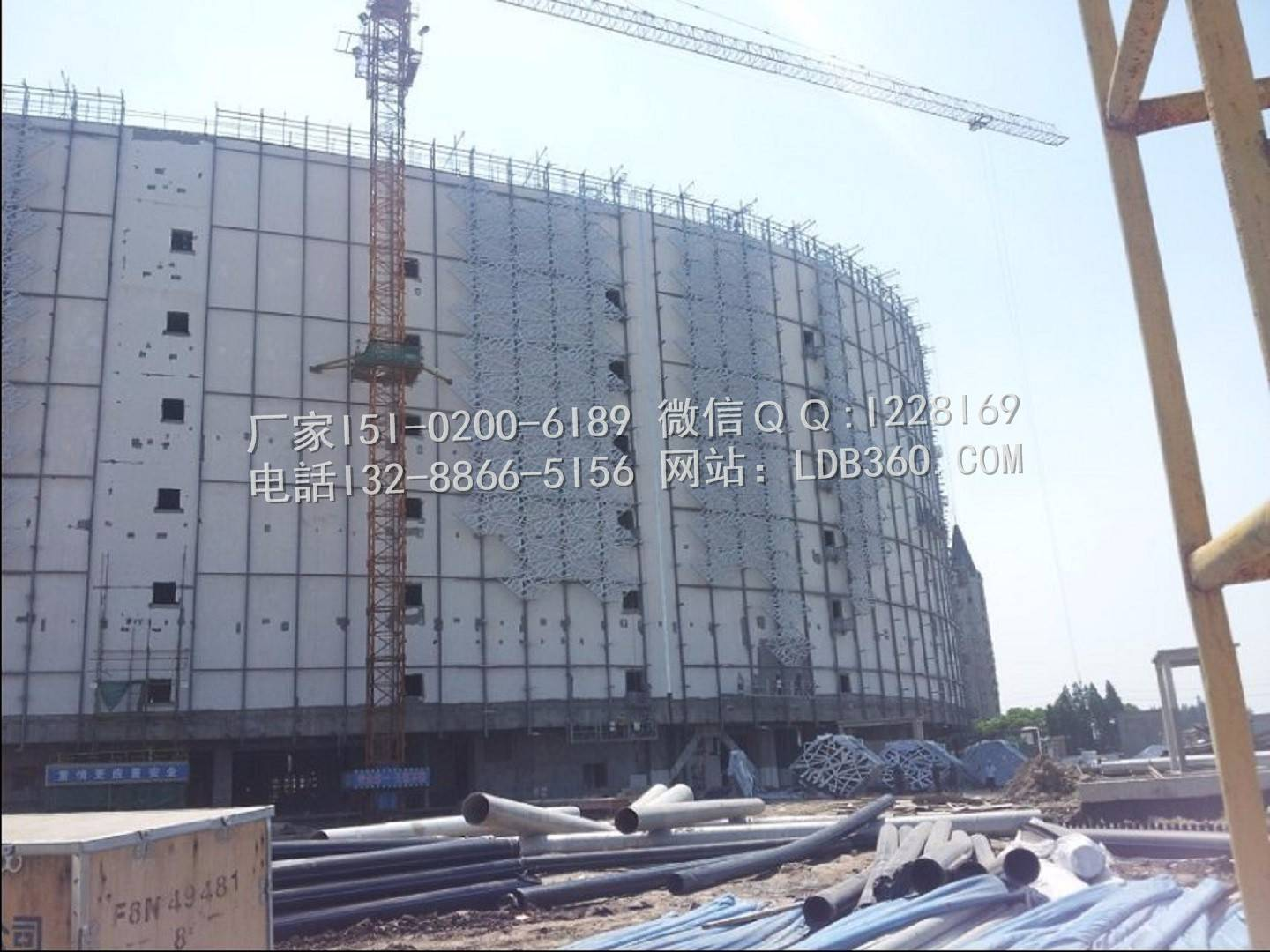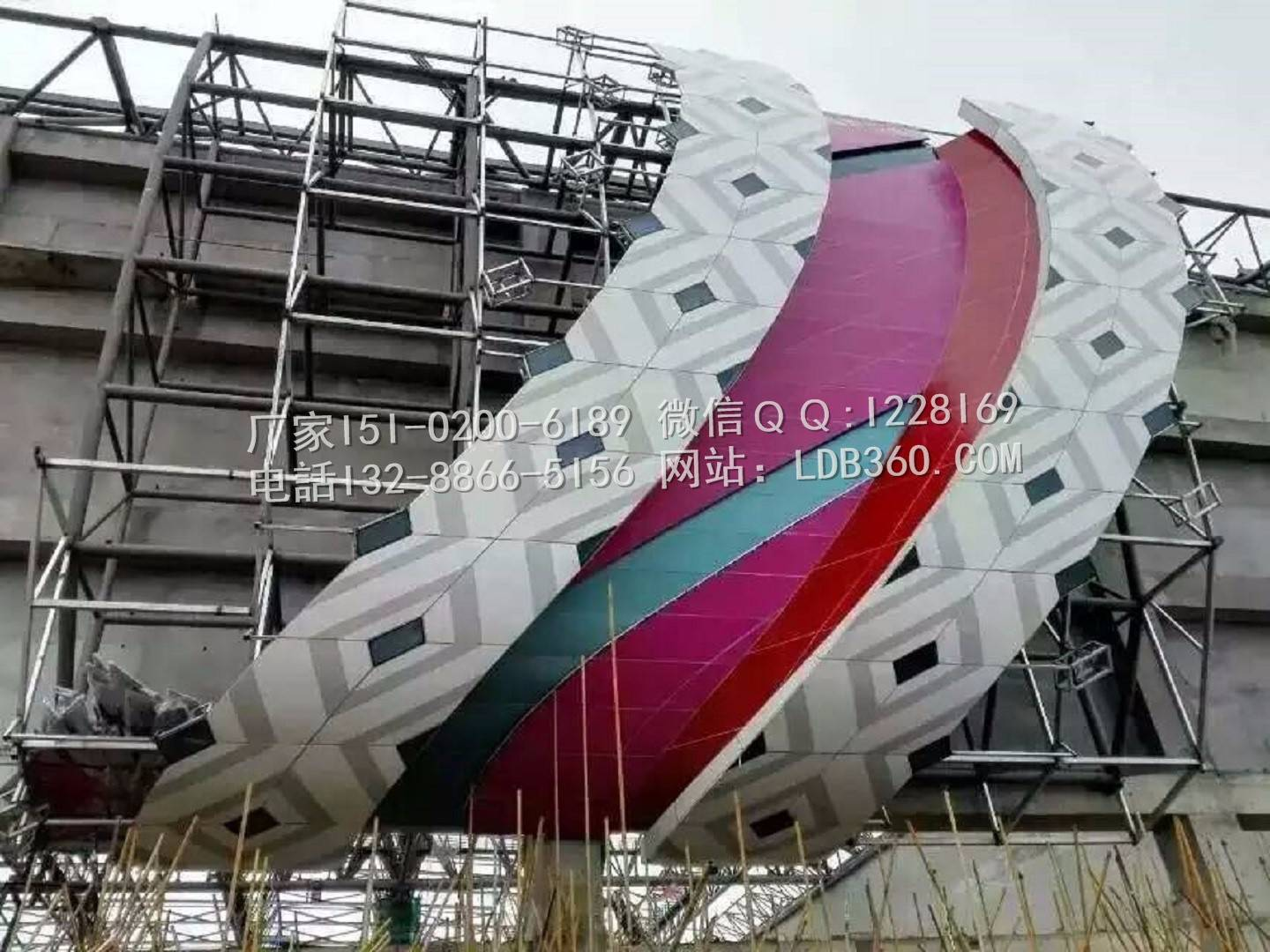# \"Metal curtain wall engineering technical code\" aluminum plate strength and deflection calculation!

2020-11-29 15:28:19 宏铝建材 5614

"Metal curtain wall engineering technical code" aluminum plate strength and deflection calculation!

Calculation conditions:

According to the position of the structural plate and the calculation object in the building, we calculate according to the most unfavorable plate. The plate adopts the four side simply supported model, the plate partition size is 1200mm (long side BA) × 600mm (short side), and the thickness of aluminum veneer is 3mm aluminum plate.

The construction elevation of the board is 20 meters, and the ground type is class C. The plate here mainly bears the gravity load, wind load and seismic load acting on the panel. The plate mainly bears the normal load of structure plane.

As the stiffness of the plate in the plane is relatively large, the effect of the plate under the in-plane load is not considered in the calculation of the plate strength and stiffness in this calculation, and the effect under the normal load is only considered as the plate and shell structure.1、 Aluminum veneer information:

Width of aluminum plate A=1200mm

Spacing between stiffeners B=600mm

Thickness of aluminum plate T=3mm

The calculation model is simply supported plate on four sides.

2、 Standard value of wind load:

According to the data you enter,

Standard value of wind load Wk =-1.2 kN/m^2

3、 Standard value of horizontal seismic action: 1

According to the data you input, the seismic intensity is 7 degrees (0.15g)

Standard value of distributed horizontal seismic action qEk=0.2 kN/m^24、 Design value of load combination:

According to: technical code for metal curtain wall engineeringJGJ133-2001Calculation formula.

The partial factor of wind load is1.4The partial coefficient of earthquake action is1.3.

The combination coefficient of wind load is1The combination coefficient of earthquake action is0.5.

q =1.4*Wk+0.5*1.3*qEk

=1.4 *-1.2+0.5*1.3*0.2

=1.836 kN/m^25、 Calculation process of stress design value

Check basis: δ=6*m*q*a^2*ETA/t^2Less than[fg]=81kN/m^2

Refer to "technical code for metal and stone curtain wall engineering"JGJ133-2001,appendixB Plate moment coefficientB.0.1

Calculation moment coefficient of aluminum plate

m=0.1022

According to "technical code for metal and stone curtain wall engineering"JGJ133-2001,The first5.4.3The fourth formula5.4.3-3

coefficient:

θ=q*a ^ 4 / (E * t ^ 4)

=1.836*600^ 4 / (0.7* 10 ^ 5 *3^ 4)

=41965.71

According to "technical code for metal and stone curtain wall engineering"JGJ133-2001surface5.4.3The first1Item:

When the deflection of the plate is greater than the thickness of the plate, the effect of large deflection should be considered in the fourth paragraph of this article, that is, the stress value multiplied by the stress reduction system.

According to θ, check "technical code for metal and stone curtain wall engineering"JGJ133-2001surface5.4.3Yes. The stress reduction factor ETA is obtained.

Stress reduction factor:ETA=0.4

Thickness of aluminum plate:t=3 mm

Short side length of aluminum plate:a=600 mm

Design value of the maximum bending stress in the plate caused by wind load and earthquake action perpendicular to the plate surface:

δ=6*m*q*a^2*η/t^2

=6*0.1022*1.836*600^ 2/3^ 2/ 1000

=45.03kN/m^2<81 kN/m^26Deflection checking calculation process of aluminum plate panel

Check basis:F=Mu*Wk*a^4*η/D Less than[Mu]=a/100=6mm

Wk =-1.2 kN/m^2

Check "technical code for metal and stone curtain wall engineering"JGJ133-2001surface5.4.3Available:

Deflection coefficient:

μ=0.00488

According to the relevant input parameters of aluminum plate, it can be calculated:

Aluminum plate stiffness:

D=70000* t^ 3 / (12 * (1 - 0.33 ^ 2))

=70000 *3^ 3 / (12 * (1 - 0.33 ^ 2))

=176747.84

According to the following coefficients:

coefficient:

θ=Wk * b0^ 4 / (7 * 10 ^ 4 * t^ 4 * 1000)

=1.2*600^ 4 / (7 * 10 ^ 4 *3^ 4 * 1000)

=27.43

According to "technical code for metal and stone curtain wall engineering"JGJ133-2001surface5.4.3The first5Item:

When calculating the deflection of the plate, the influence of large deflection should also be considered, and the deflection value calculated according to the small deflection formula should also be multiplied by the reduction coefficient.

Reduction factor:

η=0.84

Deflection of aluminum plate:

f=μ* Wk * b0 ^ 4 * η / ( D * 1000)

=0.00488*1.2*600^ 4 *0.84343/ (176747.84* 1000)

=3.62mm<6mm7、 The calculation results are as follows

Aluminum plate strength and deflection meet the requirements of the specification!